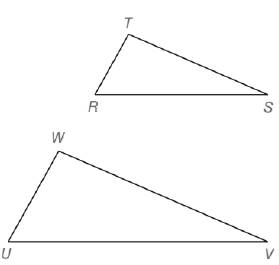Chapter 5.CT, Problem 5CTElementary Geometry For College St...

7th Edition
Alexander + 2 others
ISBN: 9781337614085

Solutions

Chapter
SectionElementary Geometry For College St...

7th Edition
Alexander + 2 others
ISBN: 9781337614085
Textbook Problem

Give the reason (AA, SAS˜, or SSS˜) why △ R T S ~ △ U T W .a) ∠ R ≅ ∠ U and T R W U = R S U V b) ∠ S ≅ ∠ V ;   ∠ T   a n d   ∠ W are right angles.To determine

a)

To reason:

RTS~UWV

Explanation

Given, RU and

TRWU=RSUV

By

To determine

b)

To reason:

RTS~UWV.

Still sussing out bartleby?

Check out a sample textbook solution.

See a sample solution

The Solution to Your Study Problems

Bartleby provides explanations to thousands of textbook problems written by our experts, many with advanced degrees!

Get Started

Under what circumstances is a t statistic used instead of a z-score for a hypothesis test?

Essentials of Statistics for The Behavioral Sciences (MindTap Course List)

The area of the region at the right is:

Study Guide for Stewart's Multivariable Calculus, 8th

Determine the size of l.

Mathematics For Machine Technology